# Module 8: Loops

## Lesson 8.3 – break and continue Commands

After reading this lesson, you should be able to:

• use the continue command in loops,

• use the break command in loops,

• decide between the use of the continue and break commands.

## What are the break and continue commands?

The break and continue commands are a way to manipulate both while and for loops, while they are running. Given this, both of these commands should always be placed inside conditional statements. This fact will become clear after reviewing the examples in this lesson.

Neither command, once it has been called, allows the current loop iteration to complete (i.e., the rest of the loop body is not going to be executed for that iteration). The commands run exactly where they are placed in the code: not at the end of the loop iteration.

## How does the break command work in MATLAB?

The break command in MATLAB is used to break out of a for or while loop; that is, it terminates the execution of the loop.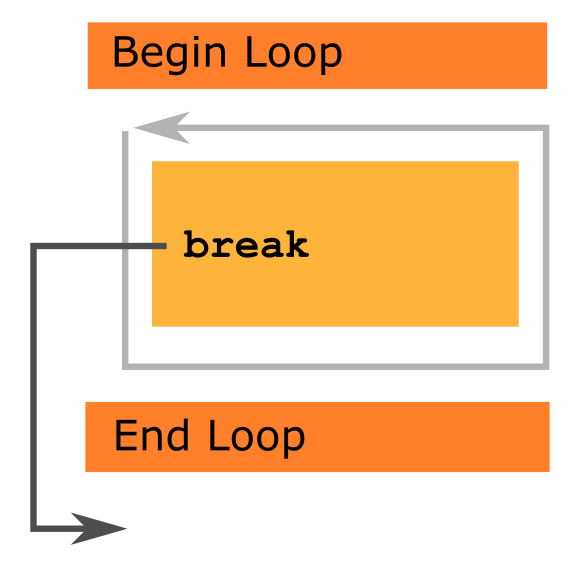Figure 1: Shows the path of execution when the break command executes.

The break command should always be used in conjunction with a conditional statement. Once the condition is true, the break command is active and the loop breaks. After the break command has terminated the loop, the segments of body code below the break command will not run. The m-file will continue to run as normal below the loop end statement. This process is visually summarized in Figure 1.

### Example 1

Write a program that calculates the values of $$k^2 - 50$$ for all integers in $$\left [ -10, 10 \right ]$$ domain, but only until $$k^2- 50$$ becomes negative. Do not continue calculating values after a negative result has been found.

Solution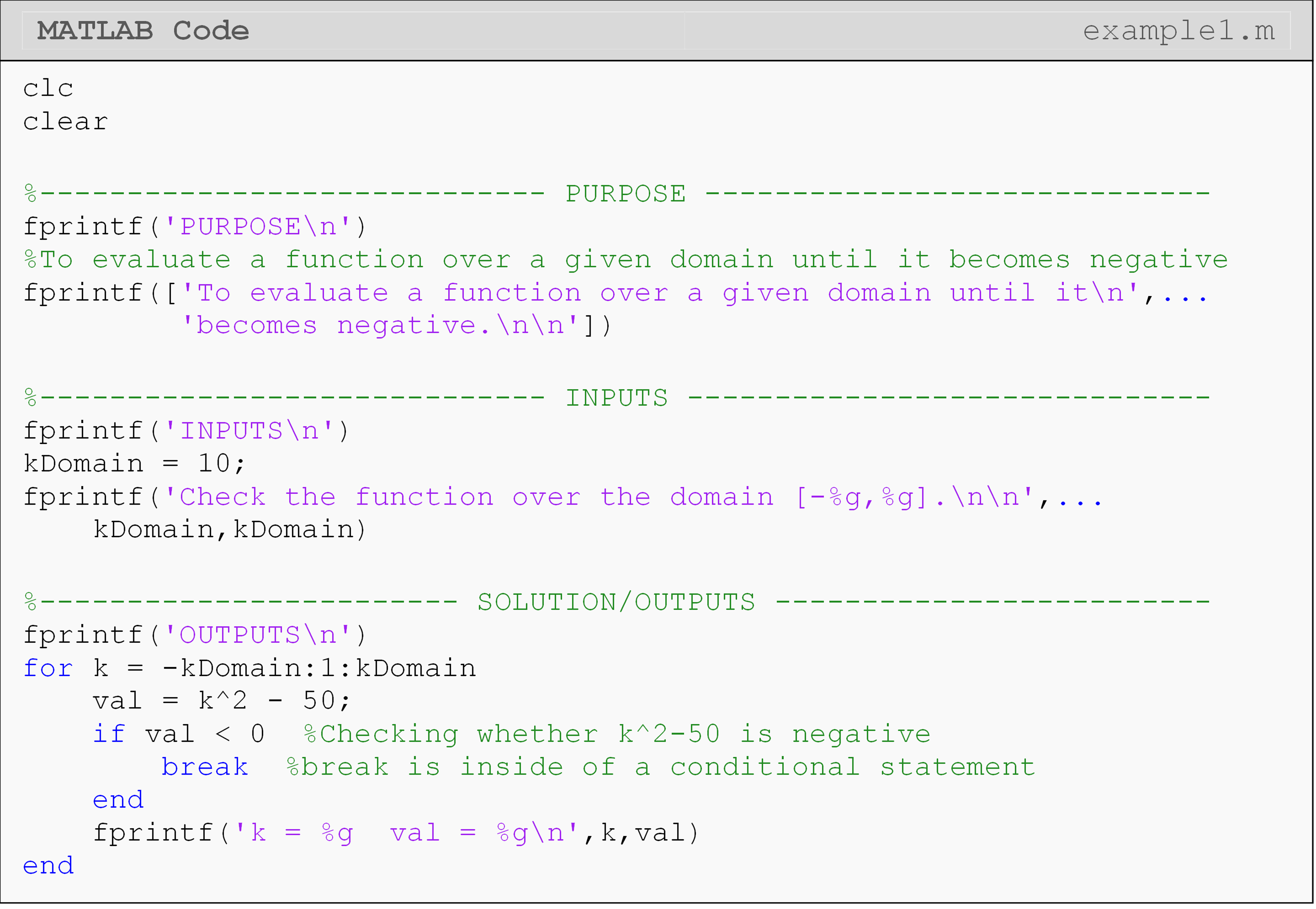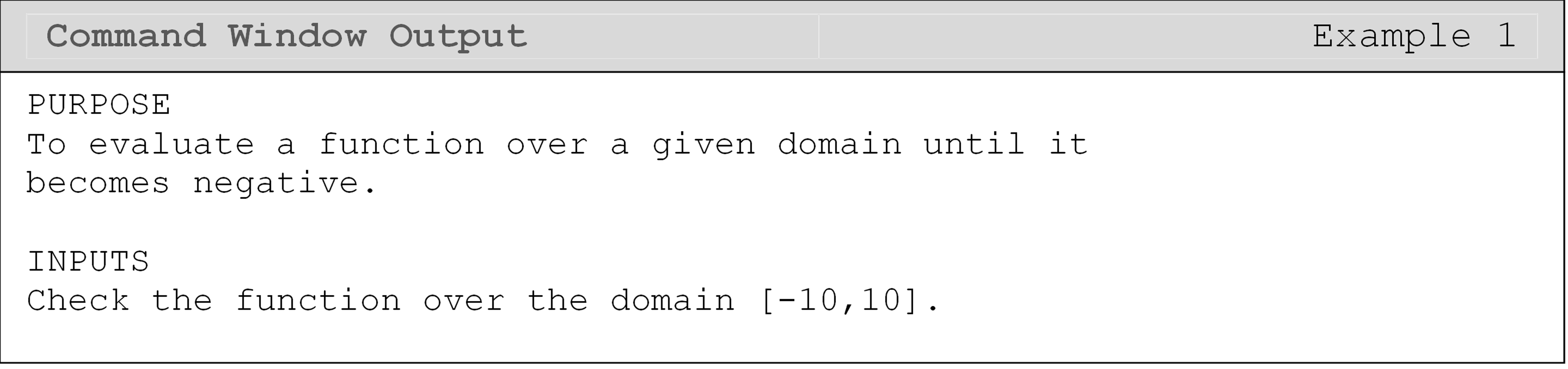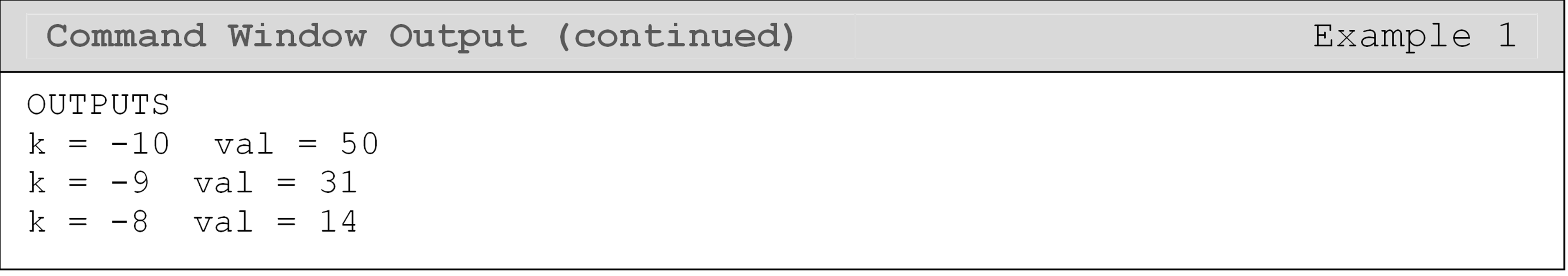Figure 2 shows the program flow when/if the break command is executed (line 21). Note the current iteration of the loop does not finish (the message on line 23 is not displayed), and the loop exits immediately. MATLAB then continues to execute the code after line 24 normally.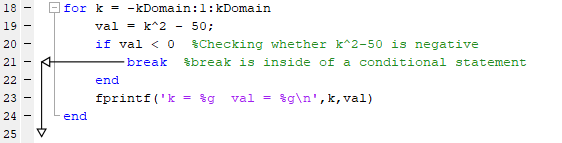Figure 2: Programming flow once the break command executes.

## How does the continue command work in MATLAB?

The continue command in MATLAB is used to conditionally pass control to the next repetition in loops (both for and while loops). Like the break command, the continue command should be used with a conditional statement. When the conditional statement is true, the continue command initiates the next loop cycle, regardless of the code in the body of the loop. The lines of code below the continue command are not run until the continue command is inactive.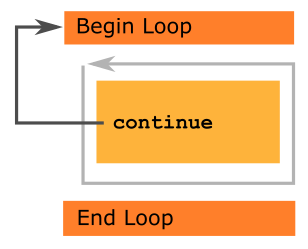Figure 3: Shows the path of execution when the continue command executes.

### Example 2

You are asked to calculate and print the values of $$k^2 - 50$$ for all integers in $$\left [ -10, 10 \right ]$$ domain, but only if $$k^2 - 50$$ is positive.

Solution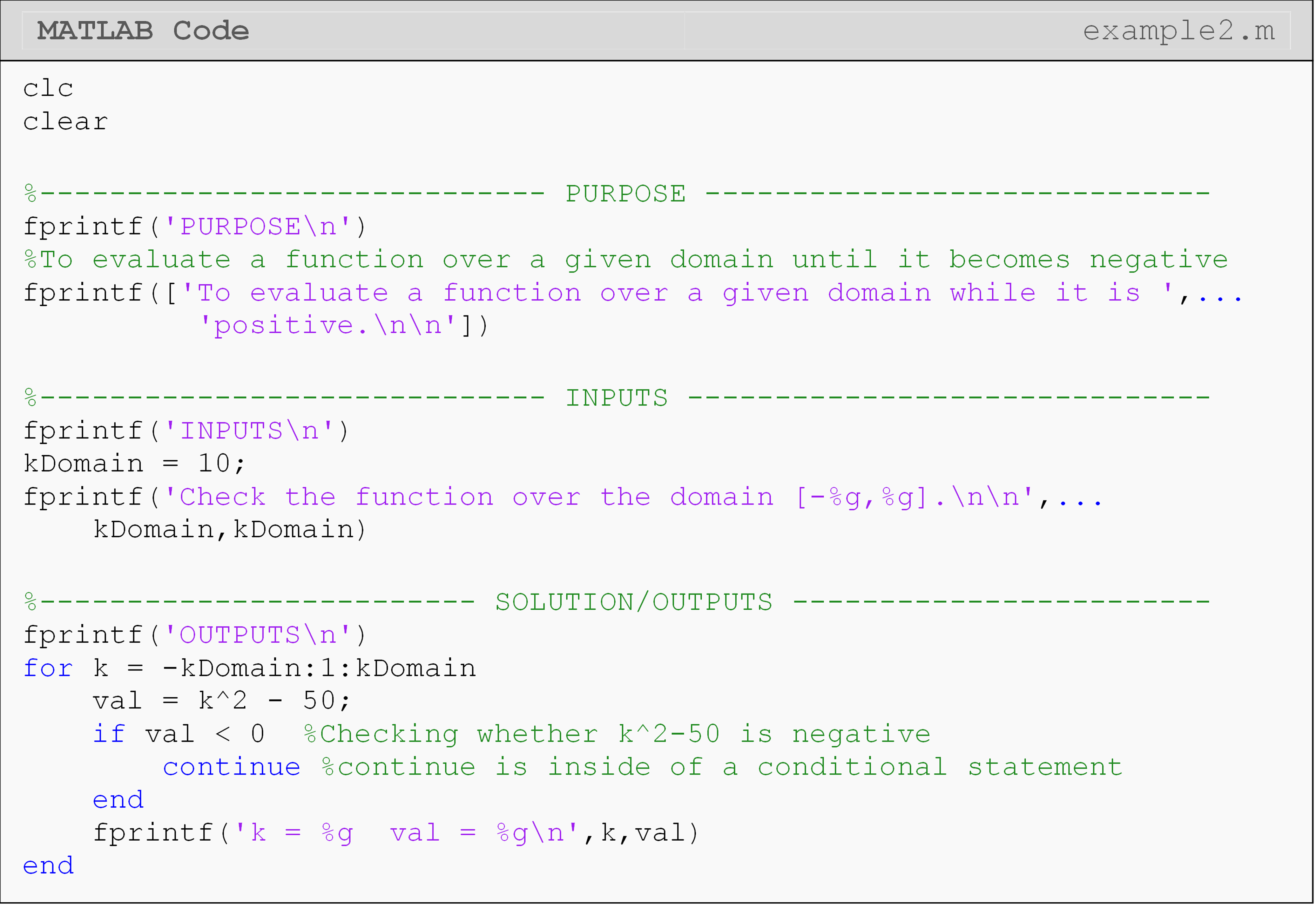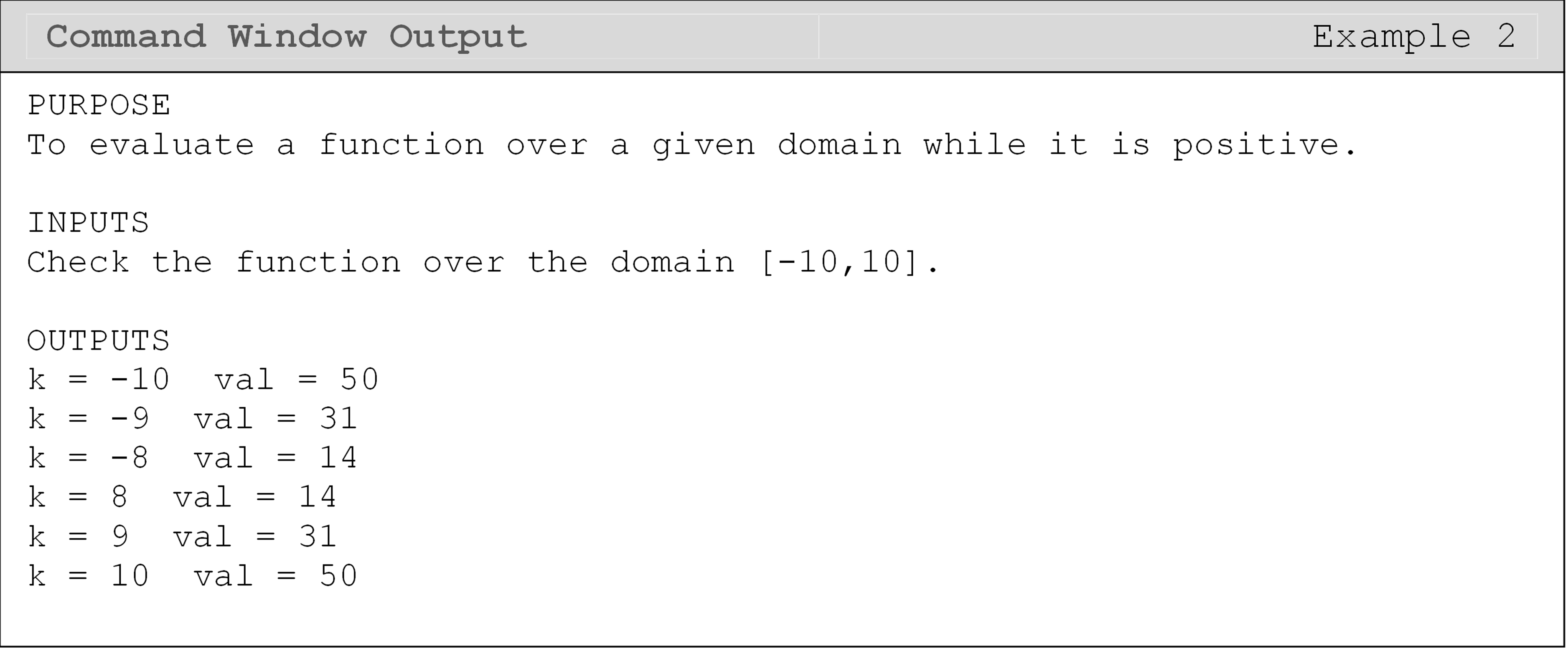Whenever the continue command becomes active, the execution jumps to the for statement of the for loop, where $$k$$ gets incremented.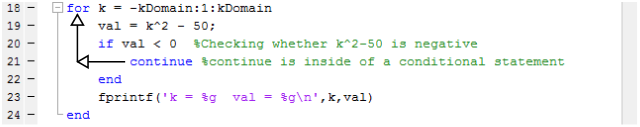Figure 4: Program flow when continue command executes.

In Figure 4, we can see that each time the continue command executes (line 21), the program immediately (without executing the rest of the loop body) continues to the next iteration of the loop. This means that the message we display with fprintf() (line 23) will only display (execute) for positive calculated numbers.

## Lesson Summary

Exit a loop before it finishes. break break
Continue to the next iteration of a loop before the current iteration finishes. continue continue

## Problem Set

(1).Use for loop(s) to write a MATLAB program that displays the value of $$r$$ and $$w$$, where

$w = - 7r^{2} + 3r + 25$

for $$r$$ in the domain [0,40] in steps of 2 units until $$w$$ turns negative.

(2). Use for loop(s) to write a MATLAB program that outputs all the negative values of $$7\sin(x)$$ in the $$x$$ domain of $$[0, 3\pi]$$. Use an interval of $$\displaystyle\frac{\pi}{10}$$.

(3). Repeat Exercise 2 with a while loop.

(4). Using a for loop, write a MATLAB function that finds the voltage ($$V$$) measured across a resistor of resistance ($$R$$) when a variable current ($$i$$) is applied to the resistor. The current is a function of time and is given by

$i(t) = 2t^{2} - 3t$

where i is measured in amperes and t in seconds. The voltage across the resistor is given by

$V = iR.$

The resistor ($$R$$) has a constant value of 120 Ω. Find the value of the voltage in the $$t$$ domain of [0, 20] in increments of 0.1. End finding the voltage when the voltage reaches 4V or more. Output the final value of the voltage and the time this value is reached.

(5). Write a MATLAB program that prompts the user to enter a vector of their choosing and storing the vector as, userVec. Via a for loop, the vector is entered one element at a time by the user through an input() prompt until the user enters over to denote the end of the vector. The program should stop prompting and display the entire vector in the Command Window.

Hint: You will have to use the vector element notation to complete this problem.

(6). Repeat Exercise 5 using the while loop.